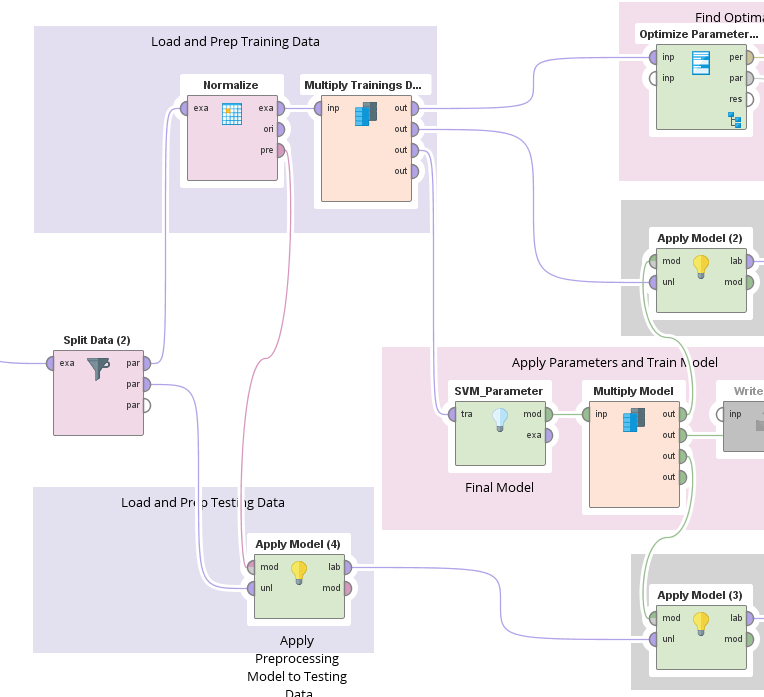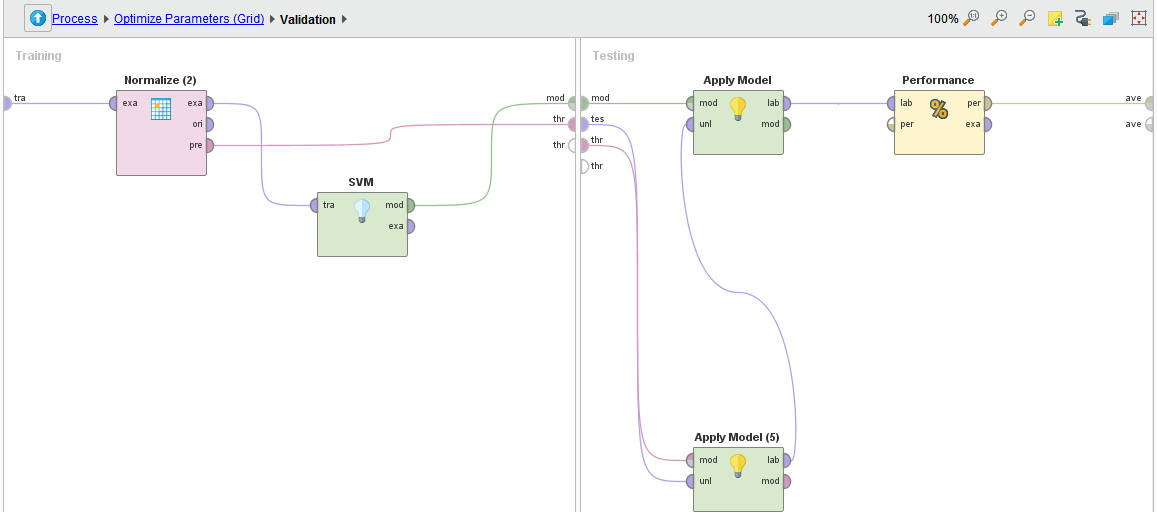# How to use standardization /normalization correctly on test/Train data set?

Member Posts: 344Unicorn
edited November 2018 in Help

hi,

I read that norm./standardization should be applied to train set separately, then the preprocessing model of the normalization/std. should be applied to the test data set,

but what about the validation set if I am doing cross-validation? should I also do a separate inner X-Validation normalization, where I apply the ranges of norm. from testdata in the XVal-set onto the validation set from the X-Validation?

For now, my process looks like this:I use once normalization on the outside "big" process, but inside the grid optimizer, I have a X-Validation with an SVM inside, however, I Am not applying further normalization on there, now my Question is, would it be better if my process looked like this:where I also apply normalization to the inner X-Validation validation data (or is it called the test-data?) and if so, what about the normalization of the outside big process, how should I use that normalization for my test-data on the outside, without already using it for the traindata set for X-Validation?

last question:

some people say (including my supervisor) that the test-data inside cross validation is called test-data, not validation data, and that  validation data is the separate data tested outside that is entirely independent from the other X-Validation datasets. Is it not the other way around?

• Member Posts: 344Unicorn

can nobody answer that question? normalization from traindata to testdata only or also applied to validation data?

• Administrator, Employee, Member Posts: 168RM Data Scientist

I am not sure if I understand your question, but see the attached process, This is how I have typically used preprocessing models during x-validation.

the model now has two inner models first normalization followed by the decision tree.

Please note that order of input models on group models is important

• RapidMiner Certified Analyst, RapidMiner Certified Expert, Member Posts: 1,761Unicorn

Hi Fred,

Sometimes the terms are bit confusing. Validation is the process in which you train and test the data to determine meaningfulness of your data. Cross Validation is this type of method. In order to properly validate and interpret your data, you must generate a training and test set.

I won't go into a discussion about cross validation - that's been covered here in the forum - but our X-Validation operator automatically creates a training and testing set iteratively.  Certain algos, such as k-nn, are suspectible to scaling problems, so you need to normalize the data. If you use 10 fold X-Val and a k-nn algo, you will generate 10 different k-nn models on 10 different test sets.  Therefore you need to normalize the 10 k-nn algos.

You're awfully close to achiving this in your above screen shots, but I offer a more elegant solution using the Group models operator.In my above screenshot you should see a Normalize operator connected to a k-nn algo and then Group Models operator.  The Training Data gets normlized and passed to K-nn. The Group Models operator is a handy operator that lets you apply models (pre-processing ones too) in specific order to the Testing Data set. In the above case, the pre-processing model for the normalization of the Training Data gets passed first to the Testing Set and hten the trained k-nn model is applied and tested for performance.

Why the heck do we do that?  The Normalization Operator comes with a default parameter set to Z-normalization. Z-normalization is the normalization technique use to generate a zero mean and 1 std dev on your training data. This pre-processed model is then passed to the testing data to make it into a zero mean 1 std dev test set so you can compare the means.  If you didn't use the z-normalization and just a regualar one, you risk the chance of have a different mean in your training and test set, thereby not honestly evaluating your data.

Hope this helps.

• Member Posts: 344Unicorn

this is even more confusing to me... so this group models is basically just passing the first model first to the testing set (and why not to the apply model operator??)

and the second model is then passed to the apply model model input...

why's it not the other way around? because I think the testing input on the other half window is coming second...?

but nonetheless, it should be just the same as my normalization process or not? Its just more explicit..

moreover, when I am doing this method, I am getting quite bad performances 70% as opposed to 84% previously..??

• Administrator, Moderator, Employee, RapidMiner Certified Analyst, RapidMiner Certified Expert, University Professor Posts: 3,453RM Data Scientist

Fred,

i think you do not get the group model operator. The group model operator creates a list of models. So you have something like:

grouped_model = [Normalization, k-NN]

if you apply the grouped model you apply the models after another. So you apply first the normalization, than the k-nn. That's why you move both over to the other side and the same normalization equation is used on the testing side.

~martin

- Sr. Director Data Solutions, Altair RapidMiner -
Dortmund, Germany
• Member Posts: 1Contributor I

So a preferred name for this operator should be "Sequence of models", right ?

• RapidMiner Certified Analyst, RapidMiner Certified Expert, Member Posts: 1,761Unicorn

Kinda maybe. What the Group Models does is great a group of pre-processed models in the training side so you can apply them on the test side.

You could still do it the old hardcore way where you add the normalization operator on the training side before the learner and then pass the PRE port via the THR port to the Testing side. Then you'll need two Apply Models operators.

This all got messy like so:

`<?xml version="1.0" encoding="UTF-8"?><process version="7.4.000">  <context>    <input/>    <output/>    <macros/>  </context>  <operator activated="true" class="process" compatibility="7.4.000" expanded="true" name="Process">    <process expanded="true">      <operator activated="true" class="x_validation" compatibility="5.1.002" expanded="true" height="124" name="Validation" width="90" x="380" y="34">        <parameter key="sampling_type" value="2"/>        <process expanded="true">          <operator activated="true" class="normalize" compatibility="7.4.000" expanded="true" height="94" name="Normalize" width="90" x="112" y="120"/>          <operator activated="true" class="k_nn" compatibility="7.4.000" expanded="true" height="76" name="k-NN" width="90" x="246" y="30">            <parameter key="k" value="11"/>          </operator>          <connect from_port="training" to_op="Normalize" to_port="example set input"/>          <connect from_op="Normalize" from_port="example set output" to_op="k-NN" to_port="training set"/>          <connect from_op="Normalize" from_port="preprocessing model" to_port="through 1"/>          <connect from_op="k-NN" from_port="model" to_port="model"/>          <portSpacing port="source_training" spacing="0"/>          <portSpacing port="sink_model" spacing="0"/>          <portSpacing port="sink_through 1" spacing="0"/>          <portSpacing port="sink_through 2" spacing="0"/>        </process>        <process expanded="true">          <operator activated="true" class="apply_model" compatibility="7.1.001" expanded="true" height="76" name="Apply Model (2)" width="90" x="45" y="75">            <list key="application_parameters"/>          </operator>          <operator activated="true" class="apply_model" compatibility="7.1.001" expanded="true" height="76" name="Apply Model" width="90" x="246" y="30">            <list key="application_parameters"/>          </operator>          <operator activated="true" class="performance" compatibility="7.4.000" expanded="true" height="76" name="Performance" width="90" x="380" y="30"/>          <connect from_port="model" to_op="Apply Model" to_port="model"/>          <connect from_port="test set" to_op="Apply Model (2)" to_port="unlabelled data"/>          <connect from_port="through 1" to_op="Apply Model (2)" to_port="model"/>          <connect from_op="Apply Model (2)" from_port="labelled data" to_op="Apply Model" to_port="unlabelled data"/>          <connect from_op="Apply Model" from_port="labelled data" to_op="Performance" to_port="labelled data"/>          <connect from_op="Performance" from_port="performance" to_port="averagable 1"/>          <portSpacing port="source_model" spacing="0"/>          <portSpacing port="source_test set" spacing="0"/>          <portSpacing port="source_through 1" spacing="0"/>          <portSpacing port="source_through 2" spacing="0"/>          <portSpacing port="sink_averagable 1" spacing="0"/>          <portSpacing port="sink_averagable 2" spacing="0"/>        </process>      </operator>      <portSpacing port="source_input 1" spacing="0"/>      <portSpacing port="sink_result 1" spacing="0"/>    </process>  </operator></process>`

This was solved by the Group Models operator clean things up. The same process above gets cleaner below:

`<?xml version="1.0" encoding="UTF-8"?><process version="7.4.000">  <context>    <input/>    <output/>    <macros/>  </context>  <operator activated="true" class="process" compatibility="7.4.000" expanded="true" name="Process">    <process expanded="true">      <operator activated="true" class="x_validation" compatibility="5.1.002" expanded="true" height="124" name="Validation" width="90" x="380" y="34">        <parameter key="sampling_type" value="2"/>        <process expanded="true">          <operator activated="true" class="normalize" compatibility="7.4.000" expanded="true" height="103" name="Normalize" width="90" x="45" y="34"/>          <operator activated="true" class="k_nn" compatibility="7.4.000" expanded="true" height="82" name="k-NN" width="90" x="246" y="30">            <parameter key="k" value="11"/>          </operator>          <operator activated="true" class="group_models" compatibility="7.4.000" expanded="true" height="103" name="Group Models" width="90" x="313" y="187"/>          <connect from_port="training" to_op="Normalize" to_port="example set input"/>          <connect from_op="Normalize" from_port="example set output" to_op="k-NN" to_port="training set"/>          <connect from_op="Normalize" from_port="preprocessing model" to_op="Group Models" to_port="models in 1"/>          <connect from_op="k-NN" from_port="model" to_op="Group Models" to_port="models in 2"/>          <connect from_op="Group Models" from_port="model out" to_port="model"/>          <portSpacing port="source_training" spacing="0"/>          <portSpacing port="sink_model" spacing="0"/>          <portSpacing port="sink_through 1" spacing="0"/>        </process>        <process expanded="true">          <operator activated="true" class="apply_model" compatibility="7.1.001" expanded="true" height="82" name="Apply Model" width="90" x="112" y="34">            <list key="application_parameters"/>          </operator>          <operator activated="true" class="performance" compatibility="7.4.000" expanded="true" height="82" name="Performance" width="90" x="246" y="34"/>          <connect from_port="model" to_op="Apply Model" to_port="model"/>          <connect from_port="test set" to_op="Apply Model" to_port="unlabelled data"/>          <connect from_op="Apply Model" from_port="labelled data" to_op="Performance" to_port="labelled data"/>          <connect from_op="Performance" from_port="performance" to_port="averagable 1"/>          <portSpacing port="source_model" spacing="0"/>          <portSpacing port="source_test set" spacing="0"/>          <portSpacing port="source_through 1" spacing="0"/>          <portSpacing port="sink_averagable 1" spacing="0"/>          <portSpacing port="sink_averagable 2" spacing="0"/>        </process>      </operator>      <portSpacing port="source_input 1" spacing="0"/>      <portSpacing port="sink_result 1" spacing="0"/>    </process>  </operator></process>`

It does take a few minutes to wrap your head around it but then it makes a lot of sense. The neat thing is that you can have both the normalize, sample, and other preprocessing operators on the training side and then apply those pre-processed models to the testing side in a very honest way with no data snooping.

• Moderator, RapidMiner Certified Analyst, Member Posts: 291Unicorn

Hi rapidminers,

I decided to follow-up on this thread as it is the closest one to my question which logically extends this topic.

So, we have validation process like this:And now we all know that all data transforms like normalization, discretizing etc has to be put INSIDE X-Validation, and here we go:This way we normalize each fold separately and also create a preprocessing model to apply to test data in each fold.

Now we have deployed the model and we expect all new data to come in and we need to score it (one by one). Something like this:Obviously, we also have to normalize the new example so it fits into the model which expects normalized data. So the question is, where do we take the preprocessing model from to normalize each new example? As soon as on the training and validation cycle we have created 10 different pre-processing models, one for each validation fold, those are valid only within their respective fold cycles, correct? But how exactly do we normalize totally new single data example we need to run thru trained, saved and deployed model?

Thanks.

• Administrator, Moderator, Employee, RapidMiner Certified Analyst, RapidMiner Certified Expert, University Professor Posts: 3,453RM Data Scientist

Hey,

keep in mind that a 10 fold x-val is running an 11th cycle. In this cycle we train a model (with preprocessing model) on the full data set. This normalization equation is used.

Cheers,

Martin

- Sr. Director Data Solutions, Altair RapidMiner -
Dortmund, Germany
• Moderator, RapidMiner Certified Analyst, Member Posts: 291Unicorn

Thanks Martin,

That nuance I totally forgotSo is it correct that we can save the preprocessing model from the latest iteration of any X-Val and use it for transforming any new example which gets into production model?